# Assignment model

2. Jun 2023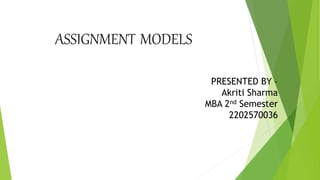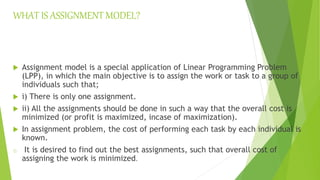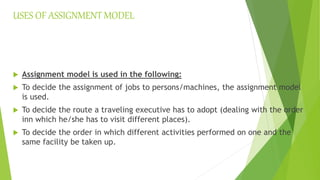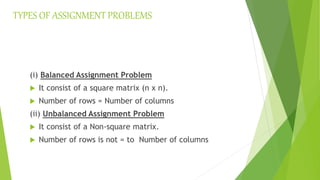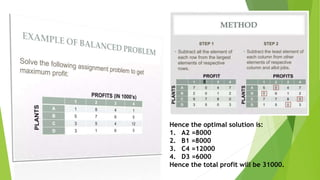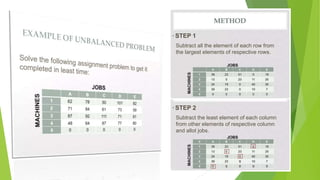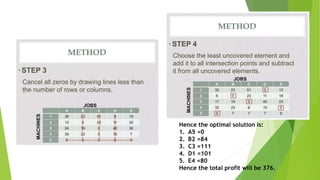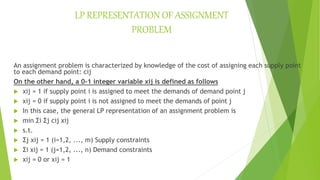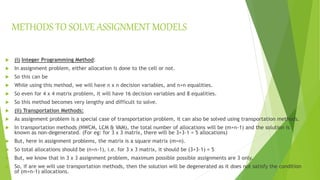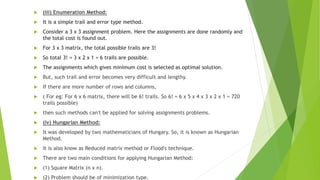1 von 11

### Assignment model

• 1. ASSIGNMENT MODELS PRESENTED BY – Akriti Sharma MBA 2nd Semester 2202570036
• 2. WHAT IS ASSIGNMENT MODEL?  Assignment model is a special application of Linear Programming Problem (LPP), in which the main objective is to assign the work or task to a group of individuals such that;  i) There is only one assignment.  ii) All the assignments should be done in such a way that the overall cost is minimized (or profit is maximized, incase of maximization).  In assignment problem, the cost of performing each task by each individual is known. o It is desired to find out the best assignments, such that overall cost of assigning the work is minimized.
• 3. USES OF ASSIGNMENT MODEL  Assignment model is used in the following:  To decide the assignment of jobs to persons/machines, the assignment model is used.  To decide the route a traveling executive has to adopt (dealing with the order inn which he/she has to visit different places).  To decide the order in which different activities performed on one and the same facility be taken up.
• 4. TYPES OF ASSIGNMENT PROBLEMS (i) Balanced Assignment Problem  It consist of a square matrix (n x n).  Number of rows = Number of columns (ii) Unbalanced Assignment Problem  It consist of a Non-square matrix.  Number of rows is not = to Number of columns
• 5. . Hence the optimal solution is: 1. A2 =8000 2. B1 =8000 3. C4 =12000 4. D3 =6000 Hence the total profit will be 31000.
• 7. Hence the optimal solution is: 1. A5 =0 2. B2 =84 3. C3 =111 4. D1 =101 5. E4 =80 Hence the total profit will be 376.
• 8. LP REPRESENTATION OF ASSIGNMENT PROBLEM An assignment problem is characterized by knowledge of the cost of assigning each supply point to each demand point: cij On the other hand, a 0-1 integer variable xij is defined as follows  xij = 1 if supply point i is assigned to meet the demands of demand point j  xij = 0 if supply point i is not assigned to meet the demands of point j  In this case, the general LP representation of an assignment problem is  min Σi Σj cij xij  s.t.  Σj xij = 1 (i=1,2, ..., m) Supply constraints  Σi xij = 1 (j=1,2, ..., n) Demand constraints  xij = 0 or xij = 1
• 9. METHODS TO SOLVE ASSIGNMENT MODELS  (i) Integer Programming Method:  In assignment problem, either allocation is done to the cell or not.  So this can be formulated using 0 or 1 integer.  While using this method, we will have n x n decision variables, and n+n equalities.  So even for 4 x 4 matrix problem, it will have 16 decision variables and 8 equalities.  So this method becomes very lengthy and difficult to solve.  (ii) Transportation Methods:  As assignment problem is a special case of transportation problem, it can also be solved using transportation methods.  In transportation methods (NWCM, LCM & VAM), the total number of allocations will be (m+n-1) and the solution is known as non-degenerated. (For eg: for 3 x 3 matrix, there will be 3+3-1 = 5 allocations)  But, here in assignment problems, the matrix is a square matrix (m=n).  So total allocations should be (n+n-1), i.e. for 3 x 3 matrix, it should be (3+3-1) = 5  But, we know that in 3 x 3 assignment problem, maximum possible possible assignments are 3 only.  So, if are we will use transportation methods, then the solution will be degenerated as it does not satisfy the condition of (m+n-1) allocations.
• 10.  (iii) Enumeration Method:  It is a simple trail and error type method.  Consider a 3 x 3 assignment problem. Here the assignments are done randomly and the total cost is found out.  For 3 x 3 matrix, the total possible trails are 3!  So total 3! = 3 x 2 x 1 = 6 trails are possible.  The assignments which gives minimum cost is selected as optimal solution.  But, such trail and error becomes very difficult and lengthy.  If there are more number of rows and columns,  ( For eg: For 6 x 6 matrix, there will be 6! trails. So 6! = 6 x 5 x 4 x 3 x 2 x 1 = 720 trails possible)  then such methods can't be applied for solving assignments problems.  (iv) Hungarian Method:  It was developed by two mathematicians of Hungary. So, it is known as Hungarian Method.  It is also know as Reduced matrix method or Flood's technique.  There are two main conditions for applying Hungarian Method:  (1) Square Matrix (n x n).  (2) Problem should be of minimization type.
• 11. THANK YOU